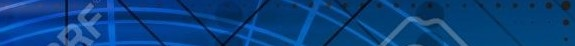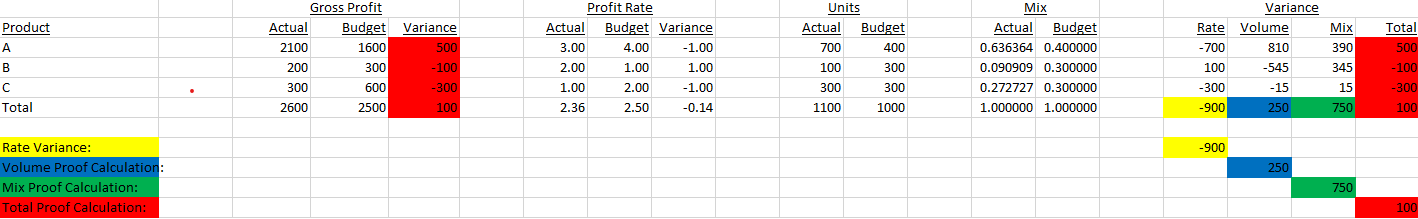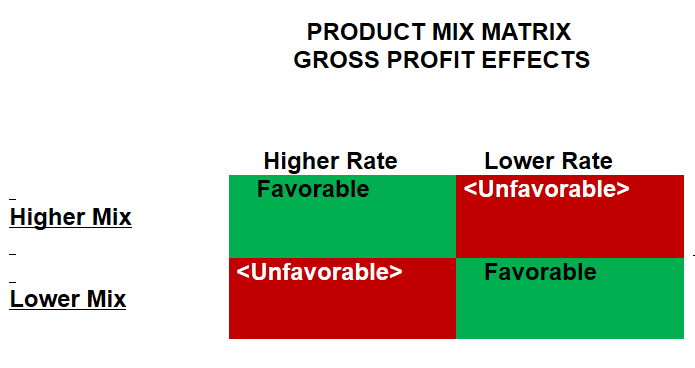# SIMPLE EXAMPLELet's analyze Product A. It is said, "If you can do one, . . . you can do them all."

Rate Variance . . .

The Rate Variance of Product A is (Actual Profit Rate minus Budget Profit Rate) multiplied by Actual Units.

Rate Variance = (3.00 - 4.00) x 700
Rate Variance = (-1.00) x 700
Rate Variance = -700

Total Variance . . .

The Total Variance of Product A (excluding Rate Variance) is (Actual Units minus Budget Units) multiplied by Budget Profit Rate.

Total Variance = (700 - 400) x 4.00
Total Variance = 300 x 4.00
Total Variance = 1200Mix Variance . . .

The Mix Variance of Product A is (Actual Mix minus Budget Mix) multiplied by (Budget Profit Rate minus Total Budget Profit Rate) multiplied by Total Actual Units.

Mix Variance = (0.636364 - 0.400000) x ( 4.00 - 2.50) x 1100
Mix Variance = 0.236364 x 1.50 x 1100
Mix Variance = 390

The simple math dynamic at the heart of Mix Variance is the delta (Budget Profit Rate minus Total Budget Profit Rate).
Any methodology which does NOT directly compare the delta of a group's aggregate profit rate with that of its' individual constituent component's profit rate is patently incorrect.

This correct methodology provides the ability to drill down to each individual constituent component's contribution to the total mix variance of its' group.

Volume Variance . . .

The Volume Variance of Product A is Total Variance minus Mix Variance.

Volume Variance = 1200 - 390
Volume Variance = 810

Note . . .

The algorithm above is applied to profit rate per sales unit. The algorithm is similarly applied to profit rate per sales currency.